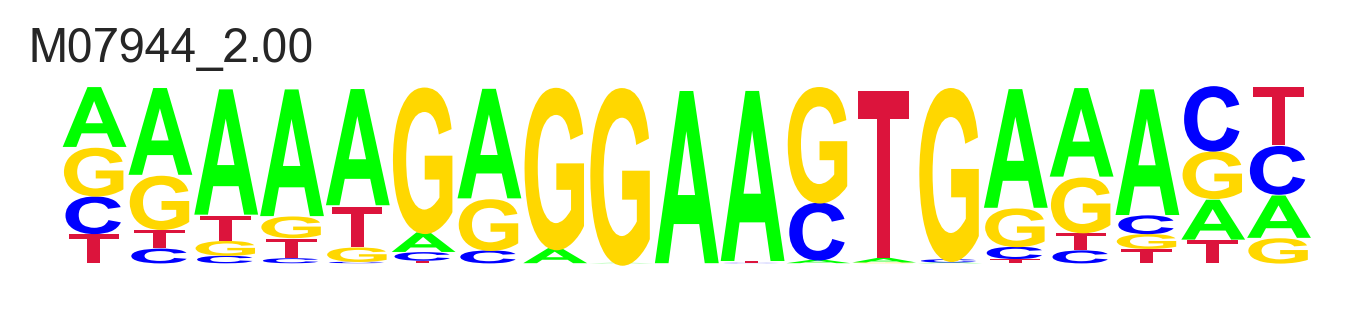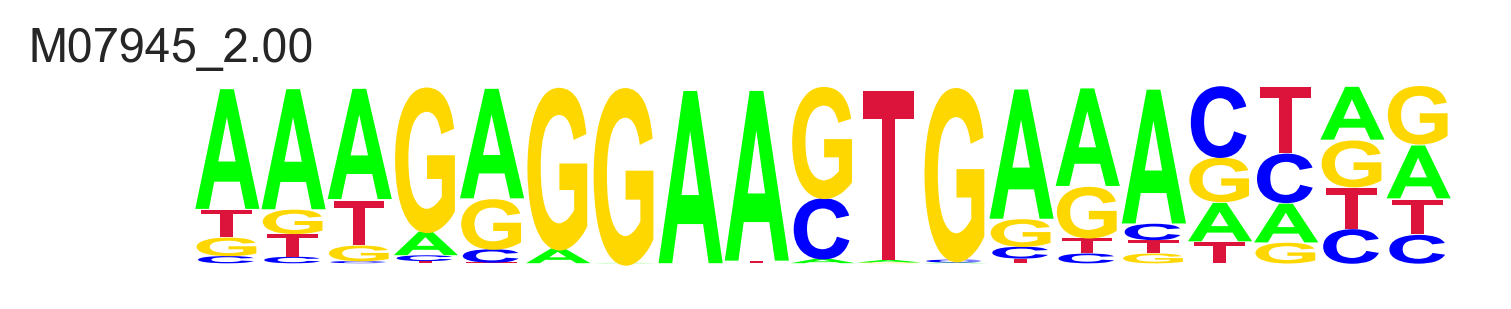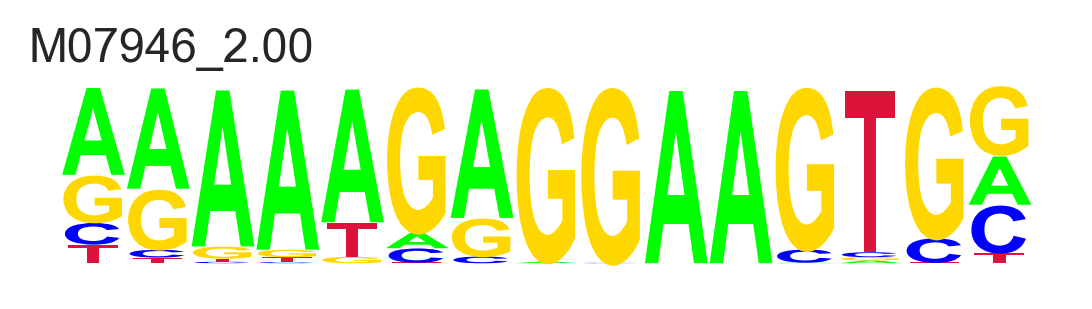## Single TF

$$\newcommand{\kon}{k_{\mathrm{on}}} \newcommand{\koff}{k_{\mathrm{off}}}$$

There exist two mathematical formalisms to describe the probabilities of molecular configurations at equilibrium: thermodynamics and kinetics. In the kinetics formalism, the system transits between configurational states according to rate parameters contained in differential equations, which can describe not just the system's equilibrium but also its trajectory towards it, or the kinetics of non-equilibrium systems. In the thermodynamics/statistical mechanics formalism, we posit that a system will occupy configurations with lower energy, and use the Boltzmann distribution to estimate the proportion of time the system spends in each state, at equilibrium. The thermodynamics formalism is limited to describing equilibrium state probabilities, but it does so with fewer parameters.

We'll derive an expression for the probability of a single TFBS' occupancy with both formalisms, but proceed with the thermodynamic description for more elaborate configurations. It will become clear why that is preferable.

### Kinetics

Most derivations of the probability of single TFBS occupancy at equilibrium employ a kinetics formalism, so we'll walk through that first, and then explore the analog in the thermodynamics description. In the kinetics description, the parameters are rates.

$$\mathrm{TF} + \mathrm{TFBS} \underset{\koff}{\overset{\kon}{\rightleftarrows}} \mathrm{TF\colon TFBS}$$

The natural rates are the rate of TF binding $\kon$ and unbinding $\koff$. Equilibrium is reached when binding and unbinding are balanced:

$$\frac{d[\mathrm{TF\colon TFBS}]}{dt} = k_{\mathrm{on}}[\mathrm{TF}][\mathrm{TFBS}] - k_{\mathrm{off}}[\mathrm{TF\colon TFBS}] = 0 \text{ at equilibrium}$$ $$k_{\mathrm{on}}[\mathrm{TF}]_{\mathrm{eq}}[\mathrm{TFBS}]_{\mathrm{eq}} = k_{\mathrm{off}}[\mathrm{TF\colon TFBS}]_{\mathrm{eq}}$$ $$\text{(dropping eq subscript) }[\mathrm{TF\colon TFBS}] = \frac{k_{\mathrm{on}}[\mathrm{TF}][\mathrm{TFBS}]}{k_{\mathrm{off}}} = \frac{[\mathrm{TF}][\mathrm{TFBS}]}{k_{d}}$$

where $k_{d} = \frac{\koff}{\kon}$ is called the dissociation constant (or equilibrium constant). We'd like to determine the probability of finding the TFBS occupied, i.e. the fraction of time it spends in the bound state. That fraction is $\frac{[\mathrm{bound}]}{([\mathrm{unbound}] + [\mathrm{bound}])} = \frac{[\mathrm{TF\colon TFBS}]}{([\mathrm{TFBS}] + [\mathrm{TF\colon TFBS}])}$. Define the denominator as $[\mathrm{TFBS}]_{0} = [\mathrm{TFBS}] + [\mathrm{TF\colon TFBS}]$ so that $[\mathrm{TFBS}] = [\mathrm{TFBS}]_{0} - [\mathrm{TF\colon TFBS}]$ and substitute:

$$[\mathrm{TF\colon TFBS}] = \frac{[\mathrm{TF}]([\mathrm{TFBS}]_{0} - [\mathrm{TF\colon TFBS}])}{k_{d}}$$ $$[\mathrm{TF\colon TFBS}](k_d + [\mathrm{TF}]) = [\mathrm{TF}][\mathrm{TFBS}]_{0}$$ $$\frac{[\mathrm{TF\colon TFBS}]}{[\mathrm{TFBS}]_{0}} = \frac{[\mathrm{TF}]}{k_d + [\mathrm{TF}]}$$

Note: We could also ask for this expression in terms of $[\mathrm{TF}]_0 = [\mathrm{TF}] + [\mathrm{TF\colon TFBS}]$ however, since we’re considering a single TFBS, $[\mathrm{TF\colon TFBS}]$ is at most 1, and so $[\mathrm{TF}]_0 \approx [\mathrm{TF}]$. In instances of ligand-receptor binding in which that approximation cannot be made, the fraction bound is a messy quadratic. Derivation here.

### Thermodynamics

In the thermodynamics description, the parameters are Gibbs free energies $\Delta G$. Let's follow the derivation from Physical Biology of the Cell (pp. 242) and consider the number microstates underlying each of the of bound and unbound macrostates, and their energies.

In order to count microstates, we imagine distributing $L$ TF molecules across a space-filling lattice with $\Omega$ sites. The energy of a TF in solution is $\varepsilon_{\mathrm{solution}}$ and the energy of a bound TF is $\varepsilon_{\mathrm{bound}}$. $\beta$ is the constant $1/k_b T$ where $k_b$ is Boltzmann's constant and $T$ is the temperature.

 State Energy Multiplicity Weight$A \cdot A_s$ $\frac{\Omega!}{(\Omega - A)!A!} \approx \frac{\Omega^{A}}{A!}$ $\frac{\Omega^{A}}{A!} \cdot e^{-\beta \left[ A \cdot A_s \right]}$$(A - 1) A_s + A_b$ $\frac{\Omega!}{(\Omega - (A - 1))!(A-1)!B!} \approx \frac{\Omega^{A-1}}{(A-1)!}$ $\frac{\Omega^{A-1}}{(A-1)!} \cdot e^{-\beta \left[ (A - 1) A_s + A_b \right]}$

In our case, the number of microstates in the unbound macrostate is $\frac{\Omega !}{L!(\Omega -L)!}\approx \frac{\Omega^L}{L!}$ and they each have energy $L \cdot \varepsilon_s$. The number of microstates in the bound macrostate is $\frac{\Omega !}{(L-1)!(\Omega -(L+1))}\approx \frac{\Omega^{(L-1)}}{(L-1)!}$ and they each have energy $(L-1) \varepsilon_s + \varepsilon_b$.

The Boltzmann distribution describes the probability of a microstate as a function of its energy: $p(E_i) = e^{-\beta E_i}/Z$ where $Z$ is the "partition function" or simply $\sum_i e^{-\beta E_i}$ the sum of the weights of the microstates, which normalizes the distribution. In our case:

$$Z(L,\Omega)=\left(\colorbox{LightCyan}{ \frac{\Omega^L}{L!} e^{-\beta L \varepsilon_s}}\right) + \left(\colorbox{Seashell}{\frac{\Omega^{(L-1)}}{(L-1)!} e^{-\beta [(L-1) \varepsilon_s + \varepsilon_b]}}\right)$$

With that expression in hand, we can express the probability of the bound macrostate, $p_b$:

$$p_b=\frac{ \colorbox{Seashell}{\frac{\Omega^{(L-1)}}{(L-1)!} e^{-\beta [(L-1) \varepsilon_s + \varepsilon_b]}}}{\colorbox{LightCyan}{\frac{\Omega^L}{L!} e^{-\beta L \varepsilon_s}} + \colorbox{Seashell}{\frac{\Omega^{(L-1)}}{(L-1)!} e^{-\beta [(L-1) \varepsilon_s + \varepsilon_b]}}} \cdot \color{DarkRed}\frac{\frac{\Omega^L}{L!}e^{\beta L \varepsilon_s}}{\frac{\Omega^L}{L!}e^{\beta L \varepsilon_s}} \color{black} = \frac{(L/\Omega)e^{- \beta \Delta \varepsilon}}{1+(L/\Omega)e^{- \beta \Delta \varepsilon}}$$

Where we have defined $\Delta \varepsilon = \varepsilon_b - \varepsilon_s$. $L/\Omega$ is really just a dimensionless TF concentration, which we'll hand-wave as being equivalent to $[\mathrm{TF}]$, which leaves us with an expression suspiciously similar to the one we derived from the kinetics formalism:

$$p_b = \frac{[\mathrm{TF}]e^{-\beta \Delta \varepsilon}}{1+[\mathrm{TF}]e^{-\beta \Delta \varepsilon}} \cdot \color{DarkRed}\frac{e^{\beta \Delta \varepsilon}}{e^{\beta \Delta \varepsilon}} \color{black} = \frac{[\mathrm{TF}]}{e^{\beta \Delta \varepsilon}+[\mathrm{TF}]}$$

From which we recapitulate an important correspondence between kinetics and thermodynamics at equilibrium: $k_d = e^{\beta \Delta \varepsilon} = e^{\Delta \varepsilon / k_bT}$ more commonly written for different units as $k = e^{-\Delta G / RT}$.

The takeaway is that both the kinetics and thermodynamics formalisms produce an equivalent expression for the probabilities of each of the bound and unbound configurations, parameterized respectively by $k_d$ and $\Delta G$.

### Sample Values

In order to compute probabilities like $p_b$, we need concrete TF concentrations $[\mathrm{TF}]$ and binding affinities (either $k_d$ or $\Delta G$). What are typical intranuclear TF concentrations and binding affinities?

#### Concentrations

A typical human cell line, K562s, have a cellular diameter of 17 microns. (BioNumbers)

def sphere_volume(d):
return 4/3*np.pi*(d/2)**3

K562_diameter_microns = 17
K562_volume_micron_cubed = sphere_volume(K562_diameter_microns)
print(f'K562 volume: {round(K562_volume_micron_cubed)} μm^3')

K562 volume: 2572 μm^3


A typical expressed TF has a per-cell copy number range from $10^3$ - $10^6$. (BioNumbers)

copy_number_range = [1e3, 1e6]
N_A = 6.02214076e23

def copy_number_and_cubic_micron_volume_to_molar_concentration(copy_number, volume=K562_volume_micron_cubed):
return (copy_number / N_A) / (volume * (1e3 / 1e18))  # 1000 Liters / m^3; 1e18 μm^3 / m^3

lower_end_molar = copy_number_and_cubic_micron_volume_to_molar_concentration(copy_number_range, K562_volume_micron_cubed)
upper_end_molar = copy_number_and_cubic_micron_volume_to_molar_concentration(copy_number_range, K562_volume_micron_cubed)

lower_end_nanomolar = lower_end_molar / 1e-9
upper_end_nanomolar = upper_end_molar / 1e-9

print('If TF copy numbers range from 1,000-1,000,000, then TF concentrations range from', str(round(lower_end_nanomolar))+'nM', 'to', str(round(upper_end_nanomolar))+'nM')

If TF copy numbers range from 1,000-1,000,000, then TF concentrations range from 1nM to 646nM


We might also like a distribution over this range. Let's posit a lognormal, where $10^3$ and $10^6$ are the 3σ from the mean, which is $10^{4.5}$. Then $\sigma = 10^{0.5}$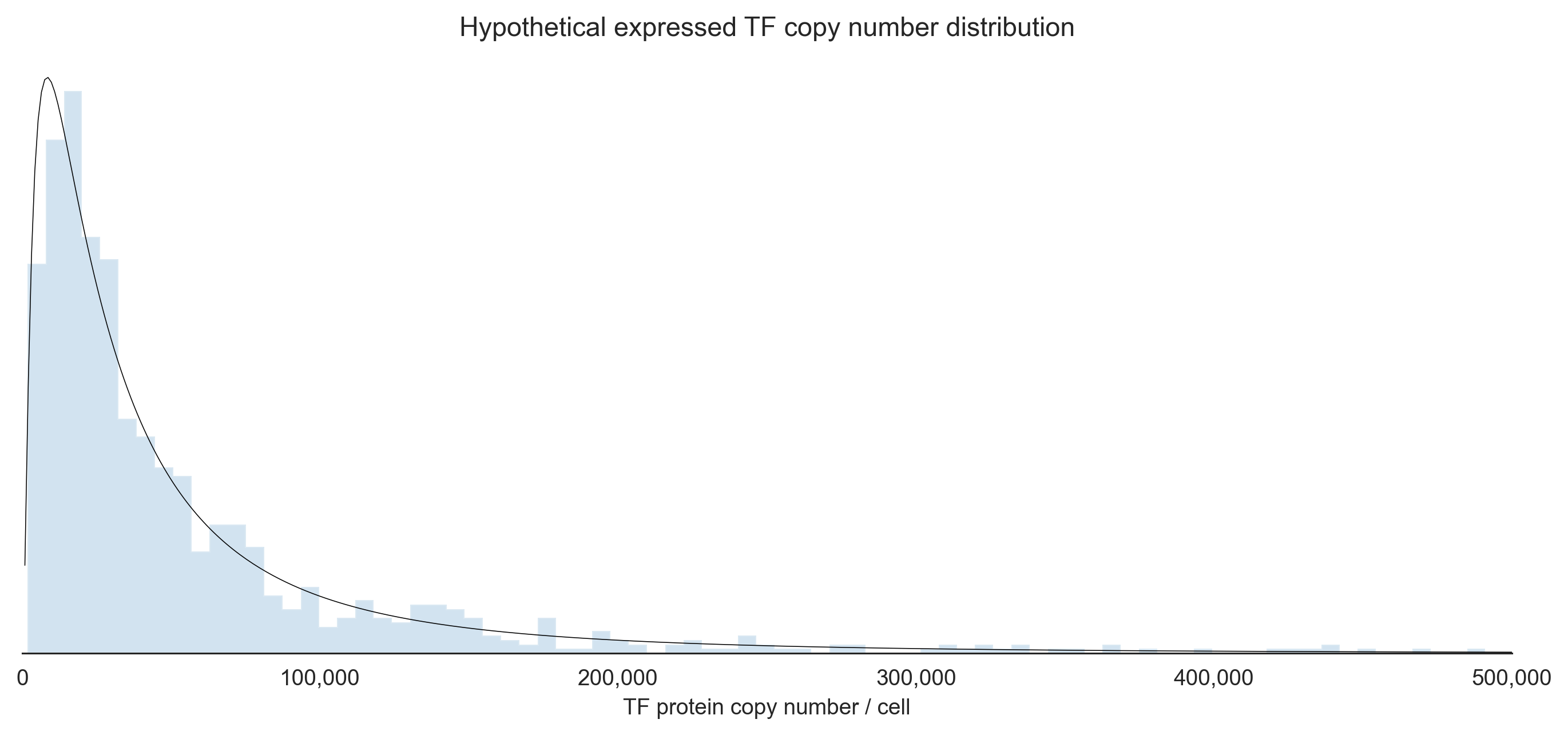#### Affinities

What are typical TF ΔG's of binding? How about the $\koff$ rates and half lives?

• We can use the prior knowledge that dissociation constants should be in the nanomolar regime (BioNumbers).
• We can use the relation that $\Delta G = -k_b T \cdot \ln(k_d)$ (Plugging in 310°K (human body temp) and the Boltzmann constant $k_b$ in kcal/Mol)
• We use the approximation that $\kon$ is ~$10^5 /$ Molar $\times$ sec (Wittrup)
T = 310
k_b = 3.297623483e-24 * 1e-3  ## cal/K * kcal/cal
kbT = k_b*T*N_A
kbT  ## in 1/Mol -- an unusual format

k_on = 1e5

def nanomolar_kd_from_kcal_ΔG(ΔG): return exp(-ΔG/kbT) * 1e9
def kcal_ΔG_from_nanomolar_kd(K_d): return -kbT*ln(K_d*1e-9)
def k_off_from_nanomolar_kd(k_d): return (k_d*1e-9) * k_on
def half_life_from_kd(k_d): return ln(2) / ((k_d*1e-9) * k_on)

$\Delta G$ $\kon$ $\koff$ $t_{1/2}$
$k_d$
1 12.757685 1e5 / (M * s) 0.0001 0 days 01:55:31.471805599
10 11.340164 1e5 / (M * s) 0.0010 0 days 00:11:33.147180560
100 9.922644 1e5 / (M * s) 0.0100 0 days 00:01:09.314718056
1000 8.505123 1e5 / (M * s) 0.1000 0 days 00:00:06.931471806

We learn that an order of magnitude residence time difference results from just 1.4 extra kcal/Mol, and that TF half lives range from about 5s to about 2h. Let's once again posit a distribution of affinities to sample from (defined on $k_d$):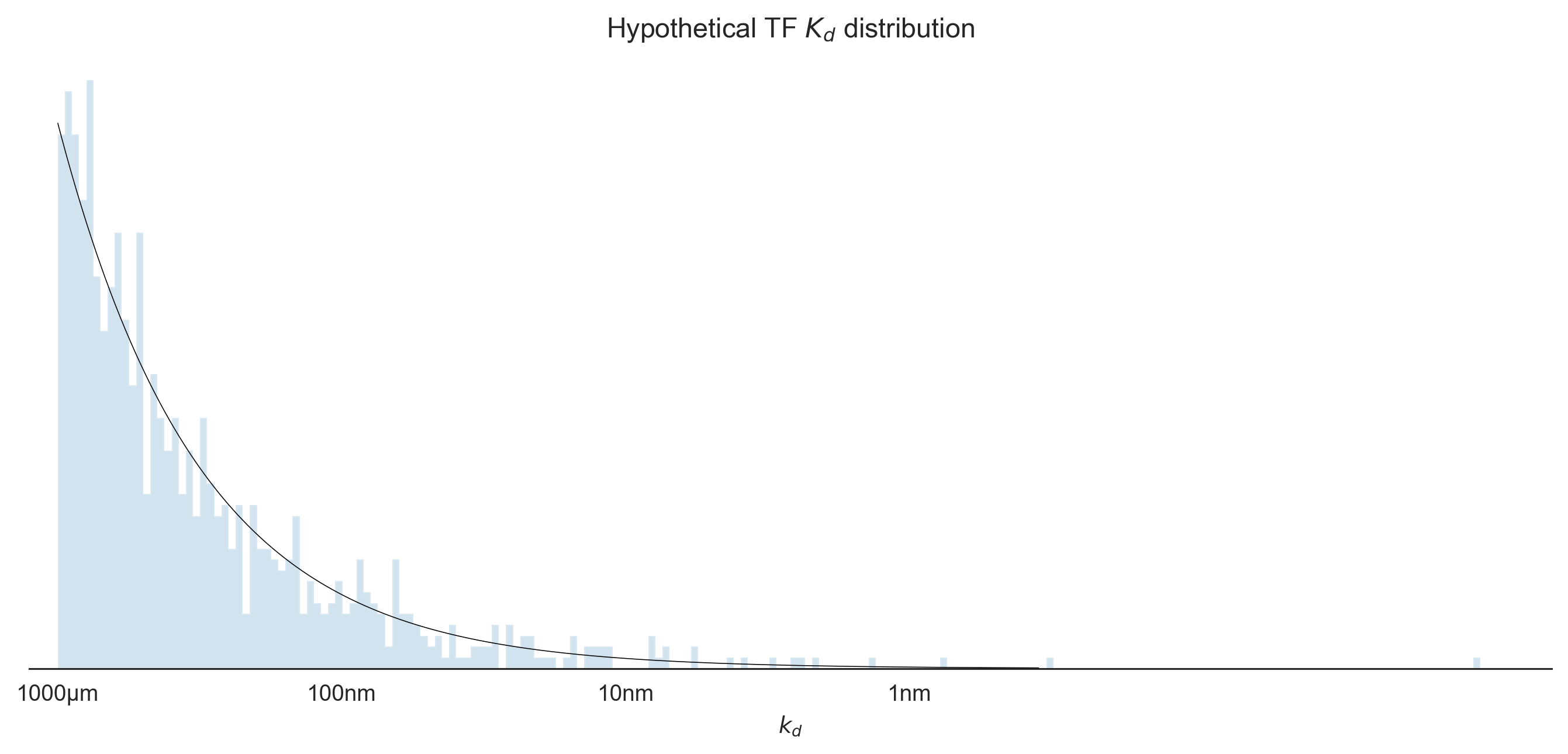With those concrete TF concentrations and dissociation constants, we can finally plot our function $p_b = \frac{[\mathrm{TF}]}{e^{\beta \Delta \varepsilon}+[\mathrm{TF}]}$.

@np.vectorize
def fraction_bound(TF, ΔG):
'''TF in nanomolar'''
return TF / (TF + nanomolar_kd_from_kcal_ΔG(ΔG))


(Note that both $[\mathrm{TF}]$ and $k_d$ are plotted in log space, but $p_b$ is linear.)

## Multiple TFs: Direct Cooperativity & Competition

Suppose now that two TFs bind adjacent segments of DNA in such a way that the binding of either facilitates (or hinders) the binding of the other. We call this direct cooperativity (and competition). We'll focus first on cooperativity.

### Cooperativity

As before, the statistical mechanics formalism entails enumerating the configurations, their multiplicities, and their energies. We'll call the TFs A and B. We'll denote their counts as $A$ and $B$. The energy of a TF in solution will once more be $A_s$ and bound to its cognate TFBS $A_b$. The energy of cooperativity will be $C_{AB}$.

 State Energy Multiplicity Weight$A \cdot A_s + B \cdot B_s$ $\frac{\Omega!}{(\Omega - A - B)!A!B!} \approx \frac{\Omega^{A+B}}{A!B!}$ $\frac{\Omega^{A+B}}{A!B!} \cdot e^{-\beta \left[ A \cdot A_s + B \cdot B_s \right]}$$(A - 1) A_s + A_b + B \cdot B_s$ $\frac{\Omega!}{(\Omega - (A - 1) - B)!(A-1)!B!} \approx \frac{\Omega^{A+B-1}}{(A-1)!B!}$ $\frac{\Omega^{A+B-1}}{(A-1)!B!} \cdot e^{-\beta \left[ (A - 1) A_s + A_b + B \cdot B_s \right]}$$A \cdot A_s + (B - 1) B_s + B_b$ $\frac{\Omega!}{(\Omega - A - (B - 1))!A!(B-1)!} \approx \frac{\Omega^{A+B-1}}{A!(B-1)!}$ $\frac{\Omega^{A+B-1}}{A!(B-1)!} \cdot e^{-\beta \left[ A \cdot A_s + (B - 1) B_s + B_b \right]}$$(A - 1) A_s + A_b + (B - 1) B_s + B_b + C_{AB}$ $\frac{\Omega!}{(\Omega - (A - 1) - (B-1))!(A-1)!(B-1)!} \approx \frac{\Omega^{A+B-2}}{(A-1)!(B-1)!}$ $\frac{\Omega^{A+B-2}}{(A-1)!(B-1)!} \cdot e^{-\beta \left[ (A - 1) A_s + A_b + (B - 1) B_s + B_b + C_{AB} \right]}$

The partition function is the sum of the weights:

$$Z = \frac{\Omega^{A+B}}{A!B!} \cdot e^{-\beta \left[ A \cdot A_s + B \cdot B_s \right]} + \frac{\Omega^{A+B-1}}{(A-1)!B!} \cdot e^{-\beta \left[ (A - 1) A_s + A_b + B \cdot B_s \right]} + \frac{\Omega^{A+B-1}}{A!(B-1)!} \cdot e^{-\beta \left[ A \cdot A_s + (B - 1) B_s + B_b \right]} + \frac{\Omega^{A+B-2}}{(A-1)!(B-1)!} \cdot e^{-\beta \left[ (A - 1) A_s + A_b + (B - 1) B_s + B_b + C_{AB} \right]}$$

Which we can greatly simplify by multiplying the entire expression by the reciprocal of the "base state" weight, $\color{DarkRed}\frac{A!B!}{\Omega^{A+B}} \cdot e^{\beta \left[ A \cdot A_s + B \cdot B_s \right]}$, normalizing that weight to 1:

$$Z = 1 + \frac{A}{\Omega} \cdot e^{-\beta \left[ A_b-A_s \right]} + \frac{B}{\Omega} \cdot e^{-\beta \left[ B_b-B_s \right]} + \frac{A \cdot B}{\Omega^2} \cdot e^{-\beta \left[ A_b-A_s+B_b-B_s+C_{AB} \right]}$$

Taking the definition $[A] = A/\Omega$ and $\Delta G_A = A_b-A_s$ produces:

$$Z = 1 + [A] e^{-\beta \left[ \Delta G_A \right]} + [B] e^{-\beta \left[ \Delta G_B \right]} + [A][B] e^{-\beta \left[ \Delta G_A+\Delta G_B+C_{AB} \right]}$$

Then the probability of any state is just the weight of that state (scaled by the weight of the base state) divided by the partition function expression $Z$.

From the above, we notice the form of the expression for the weight of a configuration of N TFBSs:

$$p_{\mathrm{config}} = \prod_{i \in \, \mathrm{bound \,TBFS}} \left( [\mathrm{TF}_{\mathrm{cognate}(i)}] \cdot e^{-\beta \left[ \Delta G_i + \sum_j c_{ij} \right]} \right) / Z$$

For numerical stability, we take the log of the unnormalized probability (that is, the weight) of configurations:

$$\log(\tilde{p}_{\mathrm{config}}) = \sum_{i \in \, \mathrm{bound \,TBFS}} \left( \log([\mathrm{TF}_{\mathrm{cognate}(i)}]) - \beta \left[ \Delta G_i + \sum_j c_{ij} \right] \right)$$

### Competition

Incorporating competition into our thermodynamic model is slightly more subtle than cooperativity, because we can imagine two types of competition

• Two TFs which may both bind DNA at adjacent sites, causing a free energy penalty due to some unfavorable interaction.
• Two TFs with overlapping DNA binding sites, which cannot physically be bound at the same time.

In the former case, the expression for $p_\mathrm{config}$ we had written before suffices, with values of $C_{AB}$ having both signs to represent cooperativity and competition. Nominally, the latter case also fits this formalism if we allow $C_{AB}$ to reach $-\infty$, but that would cause us headaches in the implementation. Instead, the weights of all those configurations which are not physical attainable, due to "strict" competitive binding between TFs vying for overlapping binding sites, are merely omitted from the denominator $Z$.

### Sample cooperativity values

In order to compute concrete probabilities of configurations, accounting for cooperativity and competition, we will need concrete energies. We'll take $C_{AB}$ to be distributed exponentially with a mean at 2.2kcal/Mol. (Forsén & Linse).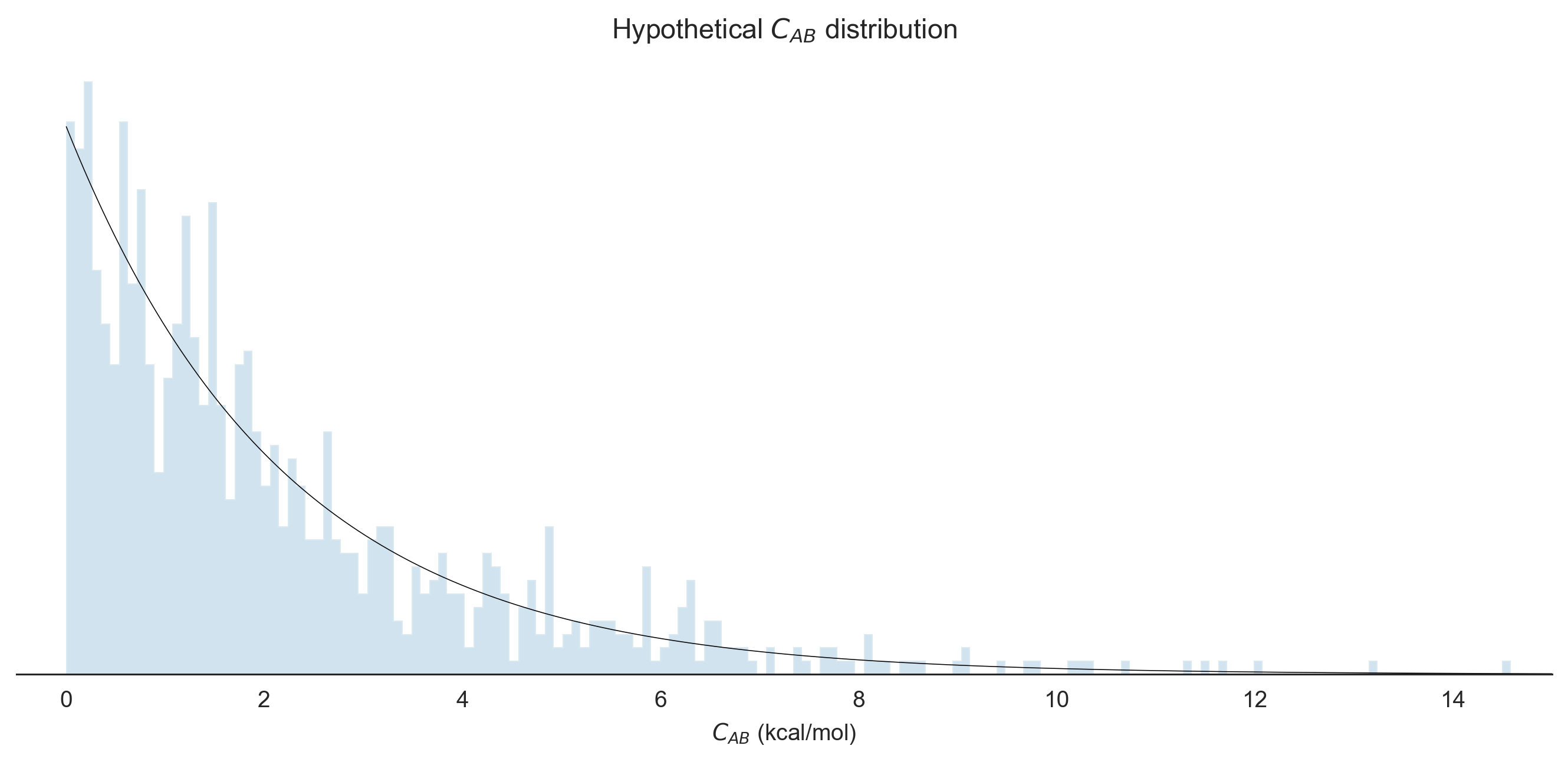Let's check our derivation (and our implementation) of $p_\mathrm{config}$ by comparing it to our direct computation of $p_b$ from §1. Single TF.

def enumerate_configs(TFBSs): return list(map(np.array, itertools.product([0,1], repeat=len(TFBSs))))

def p_configs(TFBSs, TF_conc, cooperativities):

configs = enumerate_configs(TFBSs)
weights = []

for config in configs:

weights.append(np.exp(log_P_config(config, TFBSs=TFBSs, TF_conc=TF_conc, cooperativities=cooperativities)))

return list(zip(configs, np.array(weights) / sum(weights)))

p_bound (prev method): 	 0.00921878699097193
p_config (new method): 	 [(array(), 0.9907812130090282), (array(), 0.00921878699097194)]With that sanity check, let's now consider the scenario of two transcription factors with cooperative binding, and compute the probabilities of each of the 4 configurations: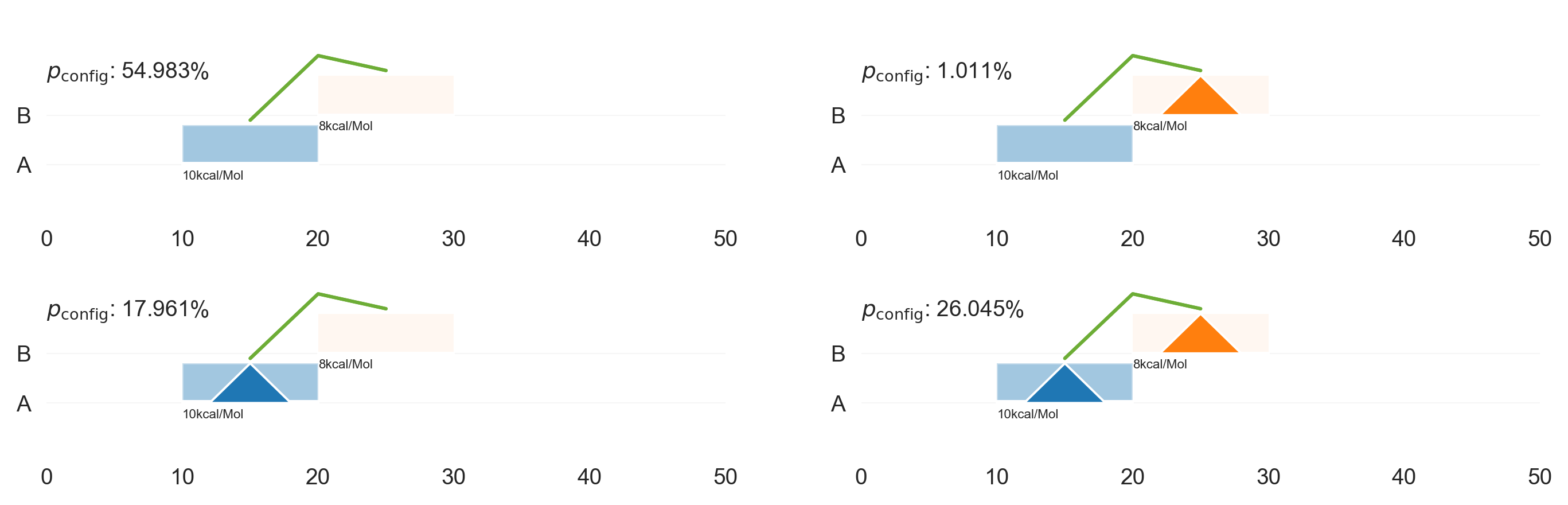## Low-Affinity binding

In reality, transcription factors' binding sites are not so discretely present or absent: transcription factors may bind anywhere along the DNA polymer, with an affinity dependent on the interaction surface provided by the sequence of nucleotides at each locus. There are a variety of approaches to model the sequence-to-affinity function for each TF. The simplest is to consider each nucleotide independently, and list the preferences for each nucleotide at each sequential position in a matrix. This type of "mono-nucleotide position weight matrix" (PWM) model is commonly used, and frequently represented by a "sequence logo" plot.

### PWMs: Construction

I have curated PWMs from various databases for ~1000 human TFs.

tfdb2 = pd.read_json('../../AChroMap/data/processed/TF.2022.json').fillna(value=np.nan)  ## prefer nan to None

probound_pwms = pd.read_json('../../AChroMap/data/processed/TF_ProBound.json', orient='records').T  # we only get probound as delta G scores, not as PWMs


As an example, consider the Transcription Factor SPI1 / PU.1, for which the following PWMs are registered in the CisBP 2.00 database:

SPI1_pwms = tfdb2.loc[(tfdb2.Name == 'SPI1')]

SPI1_cisbp_pwms = cisbp_pwms.loc[SPI1_pwms.CISBP_2_strict.iat]
SPI1_humanTFs_pwms = humanTFs_pwms.reindex(SPI1_pwms.HumanTFs.iat).dropna()
SPI1_jaspar2022_pwms = jaspar2022_pwms.loc[SPI1_pwms.JASPAR_2022.iat]
SPI1_probound_pwms = probound_pwms.loc[SPI1_pwms.ProBound.iat].pwm

for (name, SPI1_pwm), offset in zip(SPI1_cisbp_pwms.items(), (0,0,2,0,0,0)):
ax = plot_pwm(SPI1_pwm, figsize=((len(SPI1_pwm)+offset+1)*0.25,1), xtick_offset=offset)
ax.set_title(name, loc='left')